# PostgreSQL Tutorial: RANK Function

August 7, 2023

Summary: in this tutorial, you will learn how to use PostgreSQL `RANK()` function to assign a rank for every row of a result set.

## Introduction to PostgreSQL `RANK()` function

The `RANK()` function assigns a rank to every row within a partition of a result set.

For each partition, the rank of the first row is 1. The `RANK()` function adds the number of tied rows to the tied rank to calculate the rank of the next row, so the ranks may not be sequential. In addition, rows with the same values will get the same rank.

The following illustrates the syntax of the `RANK()` function:

``````RANK() OVER (
[PARTITION BY partition_expression, ... ]
ORDER BY sort_expression [ASC | DESC], ...
)
``````

In this syntax:

• First, the `PARTITION BY` clause distributes rows of the result set into partitions to which the `RANK()` function is applied.
• Then, the `ORDER BY` clause specifies the order of rows in each a partition to which the function is applied.

The `RANK()` function can be useful for creating top-N and bottom-N reports.

## PostgreSQL `RANK()` function demo

First, create a new table named `ranks` that contains one column:

``````CREATE TABLE ranks (
c VARCHAR(10)
);
``````

Second, insert some rows into the `ranks` table:

``````INSERT INTO ranks(c)
VALUES('A'),('A'),('B'),('B'),('B'),('C'),('E');
``````

Third, query data from the `ranks` table:

``````SELECT
c
FROM
ranks;
``````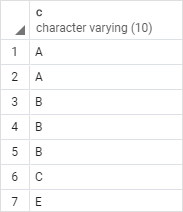Fourth, use the `RANK()` function to assign ranks to the rows in the result set of `ranks` table:

``````SELECT
c,
RANK () OVER (
ORDER BY c
) rank_number
FROM
ranks;
``````

The following picture shows the output: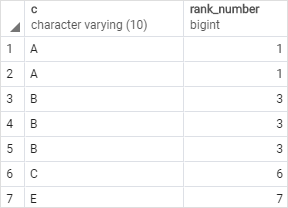As you can see clearly from the output:

• The first and second rows receive the same rank because they have the same value `A`.
• The third, fourth, and fifth rows receive the rank 3 because the `RANK()` function skips the rank 2 and all of them have the same values `B`.

## PostgreSQL `RANK()` function examples

We’ll use the `products` table to demonstrate the `RANK()` function: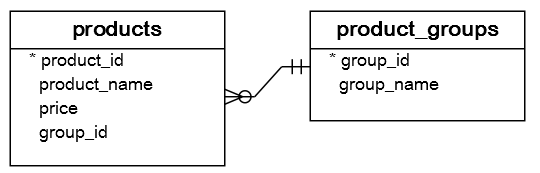This picture shows the data of the `products` table: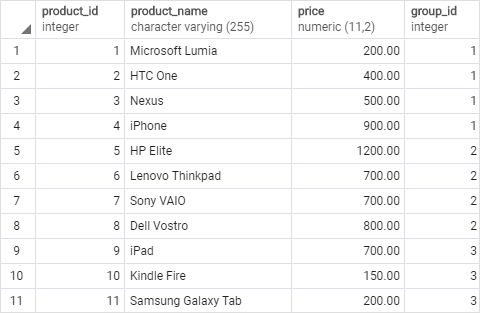### 1) Using PostgreSQL `RANK()` function for the whole result set

This example uses the `RANK()` function to assign a rank to each product by its price:

``````SELECT
product_id,
product_name,
price,
RANK () OVER (
ORDER BY price DESC
) price_rank
FROM
products;
``````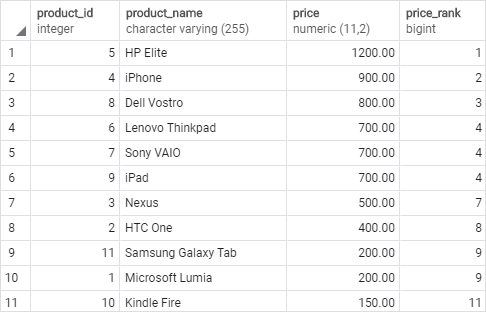In this example, we omitted the `PARTITION BY` clause, therefore, the `RANK()` function treated the whole result set as a single partition.

The `RANK()` function calculated a rank for each row within the whole result set sorted by prices from high to low.

### 2) Using PostgreSQL `RANK()` function with `PARTITION BY` clause example

The following example uses the `RANK()` function to assign a rank to every product in each product group:

``````SELECT
product_id,
product_name,
group_name,
price,
RANK () OVER (
PARTITION BY p.group_id
ORDER BY price DESC
) price_rank
FROM
products p
INNER JOIN product_groups g
ON g.group_id = p.group_id;
``````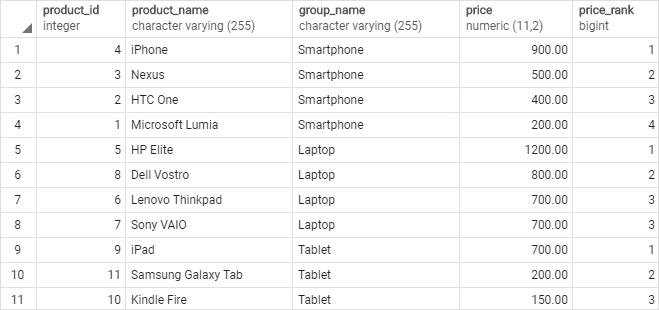In this example:

• First, the `PARTITION BY` clause distributes products into partitions grouped by product group id (`group_id`).
• Second, the `ORDER BY` clause sort products in each partition by their prices from high to low.

The `RANK()` function was applied to every product in each product group and it is reinitialized when the product group changed.

In this tutorial, you have learned how to use the PostgreSQL `RANK()` function to calculate a rank for every row in a partition of a result set.